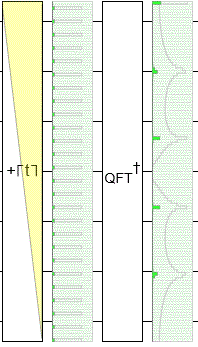# Quantum phase estimation - Question regarding rewriting the state

• I
• Peter_Newman

#### Peter_Newman

In Nielsen and Chuang p.223 we have the following situation:

$$\frac{1}{2^t} \sum\limits_{k,l=0}^{2^t-1} e^{\frac{-2\pi i k l}{2^t}} e^{2 \pi i \varphi k} |l\rangle$$

Which results from applying the inverse quantum Fourier transform to state ##\frac{1}{2^{t/2}} \sum\limits_{k=0}^{2^t-1} e^{-2\pi i \varphi k} |l\rangle##. We have more or less a sum over the basis states ##|l\rangle##. This is clear so far.

Next, the following new notation is then introduced ##|(b+l)(\text{mod } 2^t)\rangle##. Were we know from ##b## that it is an integer in the range from ##0## to ##2^t -1##.

What does this ##b+l## mean exactly, how is this to interpret? Why does one not leave it with the basic state ##|l\rangle##?

Last edited:

You have computational basis states ##|0\rangle##, ##|1\rangle##, ##|2\rangle##, etc.

Instead of writing ##|2\rangle## you could write ##|1+1\rangle## because ##1+1=2##.

Instead of writing ##|2\rangle## you could write ##|3+7 \pmod{8}\rangle## because ##(3+7) \bmod 8 = 2##.

Get the idea? From that you can see that looking at ##\sum_\ell |b+\ell \pmod{N}\rangle## instead of ##\sum_\ell |\ell\rangle## is shifting the labels of the states over by some fixed offset ##b##, wrapping around just before you get to ##|N\rangle##.

•Peter_Newman
Hello, thank you for the answer!

Yes because of the modular arithmetic one always remains in the "limits" of the index. One could also have added 42 to each state and calculate ##\text{mod } 2^t##, I suppose ;)

But what is the advantage of this? I mean, one does not introduce a new notation, in order to have nothing of it at the end?

Ah, it's not a description of a solution it's a description of a problem. When you have a register x that contains a uniform superposition and you measure f(x), where f is a periodic function period p, you are left with a superposition where every p'th state is present... with some uncontrollable offset b. The book is then going to show that when you take the Fourier transform of this register, the offset b becomes purely phase information; the magnitudes of the amplitudes of the superposition don't depend on b. Measuring in the frequency basis avoids the unknown offset problem.Image is from here: https://algassert.com/post/1718

•Peter_Newman